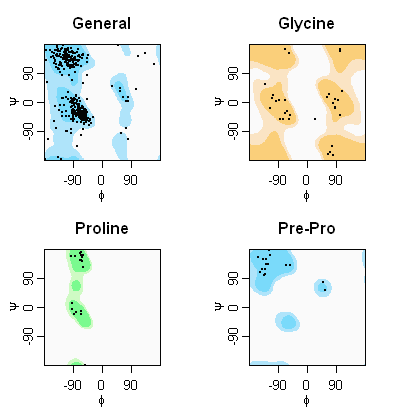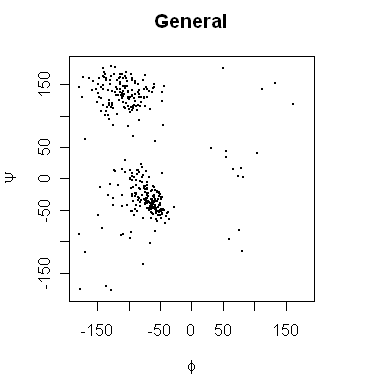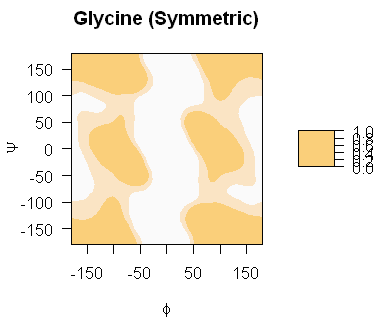# Drawing Ramachandran (phi/psi) plots for Proteins

These pages shows how to use R to draw a protein backbone's psi/phi torsion angles (ϕ,ψ) from a supplied file as a scatter plot overlayed on a filled contour plot showing the favoured and allowed regions, for example:This R example does not read PDB files to calculate the (ϕ,ψ) angles directly - you have to supply them as an input file. For example, this tab separated variable file which I created using Python from PDB file 1HMP.

The code also relies on supplied background distributions to draw its filled contour plot - these datafiles are from Lovell et al. 2003 (see references). The colour scheme used is that of their online tool RAMPAGE (see other tools/programs for Ramachandran Plots), which produces even nicer images.

My code assumes you will have an input file where each line contains one (ϕ,ψ) angle pair (between -180 and 180 degrees) with the associated "Ramachandran Type" - i.e. Glycine, Proline, Pre-Proline or General.

The example input file (1HMP_mmtk.tsv, tab separated variable file created in python) looks like this:

```1HMP:chain0:Pro5	-92.926842	12.941312	Proline
1HMP:chain0:Gly6	65.796807	-162.229709	Glycine
1HMP:chain0:Val7	-81.132082	121.413022	General
...
1HMP:chain1:Lys216	-168.783005	-116.244225	General
```

We can read this into R using the first column as the row name with one command:

Its then very easy to filter this to get just the "Glycine" pairs for example:

```> scatter.data[which(scatter.data[,"type"]=="Glycine"),"phi"]
   65.79681   79.01638  -54.12254  -56.43478   85.66304
 -109.14374 -170.14536   23.78637 -106.75558 -115.52499
   61.59938   95.28372   68.70101  -75.43935  103.26203
  -60.65742   81.68372   71.33807  -69.08505  -66.91649
  101.40772  107.48315 -131.43212  -67.83675  -95.88951
  -86.20875   86.26477   87.74782   79.22726  -83.67055
  103.86635  -55.16140
> scatter.data[which(scatter.data[,"type"]=="Glycine"),"psi"]
 -162.229709 -136.703332  -38.858768  151.516345    9.247443
   11.702525  178.494853  -52.570408   22.092137  -17.593558
   14.708826    6.490617   24.583907  -53.403879  159.510235
  -29.319241 -164.401138 -155.176242  -32.708979  165.032486
  -39.933464   32.899630   56.793570    6.169893    1.919425
    5.175914  -17.154791  -15.588814   -4.129135  -52.845410
  163.277791  -51.899098```

Using R's plot command creating a simple scatter plot is then very easy:

> scatter.psi <- scatter.data[which(scatter.data[,"type"]=="General"),"psi"]
> scatter.phi <- scatter.data[which(scatter.data[,"type"]=="General"),"phi"]
> par(pty="s")
> plot(x=scatter.phi, y=scatter.psi, xlim=c(-180,180), ylim=c(-180,180), main="General", pch=20, xlab=expression(phi), ylab=expression(psi), pch=20, cex=0.1, asp=1.0)Note that we used xlab=expression(phi) to get the Greek letter ϕ as the x-axis label. The option pch=20 gives dots (rather than the default of circles) for each datapoint, and cex=0.1 makes them smaller than by default. Finally asp=1.0 asks for an aspect ratio of one, and par(pty="s") requested a square plotting area.

The following code is written to load the rama500-*.data files from Lovell et al. 2003 (see references, and downloads). For example, their file rama500-general.data looks like this:

```# Table name/description: "Top500 General case (not Gly, Pro, or pre-Pro) B<30"
# Number of dimensions: 2
# For each dimension, 1 to 2: lower_bound  upper_bound  number_of_bins  wrapping
#   x1: -180.0 180.0 180 true
#   x2: -180.0 180.0 180 true
# List of table coordinates and values. (Value is last number on each line.)
-179.0 -179.0 0.00782923406455425
-179.0 -177.0 0.00641357067237856
-179.0 -175.0 0.005231799492823202
-179.0 -173.0 0.004234680060073368
...
179.0 179.0 0.006881355097619223```

I wrote an R function to load this data format, and turn it into an array suitable for use with R's contour functions (see downloads). It assumes that the grid is 180 by 180 in size (2 degrees per bin) with the grid points (mid points) at -179, -177, ..., to 179 degrees. For reasons of speed, it also make a big assumption about the order that the data will be found in...

Having loaded the data, you can draw it using the contour or filled.contour functions:

> par(pty="s")
> filled.contour(x=mid.points, y=mid.points, z=grid, levels=c(0,0.002,0.02,1), col=c("#FFFFFF","#FFE8C5","#FFCC7F"), main="Glycine (Symmetric)", asp=1.0, xlab=expression(phi), ylab=expression(psi))The thresholds are from the original reference, and the glycine colours are as used in RAMPAGE.

As before, we ensured a square plot area with par(pty="s") and setting the aspect ratio to one (asp=1.0). As far as I can tell, there is no way to turn off the key (which is worse the useless in this case), but all is not lost...

## Combining Scatter & Contour Plots

One of the nice things about R is that you can look at the source code to most of the built in functions - just try typing filled.contour at the R command prompt. I started with a copy of this code, removed the bits to draw the key, and added code to overlay a scatter plot to make my own function, ramachandran.plot (see downloads).

This preample code setups the filenames, thresholds, and colours for the four different plots:

```mid.points <- seq(-179,179,2)

grid.filenames <- c(General="rama500-general.data",
Glycine="rama500-gly-sym.data",
Proline="rama500-pro.data",
Pre.Pro="rama500-prepro.data")

grid.columnnames <- c(General="General",
Glycine="Glycine",
Proline="Proline",
Pre.Pro="Pre-Pro")

grid.levels <- t(cbind(General=c(0, 0.0005, 0.02, 1),
Glycine=c(0, 0.002,  0.02, 1),
Proline=c(0, 0.002,  0.02, 1),
Pre.Pro=c(0, 0.002,  0.02, 1)))

grid.colors <- t(cbind(General=c('#FFFFFF','#B3E8FF','#7FD9FF'),
Glycine=c('#FFFFFF','#FFE8C5','#FFCC7F'),
Proline=c('#FFFFFF','#D0FFC5','#7FFF8C'),
Pre.Pro=c('#FFFFFF','#B3E8FF','#7FD9FF')))

grid.dir <- "top500-angles/pct/rama/"
scatter.filename <- "1HMP.tsv"```

The following loads the example datafile, and then draws the four Ramachandran plots, using the specified four distributions loaded from their files:

```scatter.data <- load.scatter(scatter.filename)

# Split the plot into quadrants:
par(mfrow=c(2,2))

for(rama.type in names(grid.filenames)) {
# Filter the input data for this graph type
col.name = grid.columnnames[rama.type]
scatter.phi <- scatter.data[which(scatter.data[,"type"]==col.name), "phi"]
scatter.psi <- scatter.data[which(scatter.data[,"type"]==col.name), "psi"]

# Load the distribution for this graph type
grid.filename <- paste(grid.dir, grid.filenames[rama.type], sep="")

# Use small margins to make the plots nice and big, which as a
# side effect means squeezing the axes labels a bit, and specify
# a SQUARE plot area (to go with aspect ratio, asp=1)
par(mar=c(3,3,3,3), mgp=c(1.75,0.75,0), pty="s")

ramachandran.plot(scatter.phi, scatter.psi,
x.grid=mid.points, y.grid=mid.points, z.grid=grid,
plot.title=col.name,
levels=grid.levels[rama.type,],
col=grid.colors[rama.type,])
}```